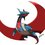# A new way to solve Quartics (Reposted)

Since I was unable to explain this properly last time,I have reposted.Hope this clears the concept. We see an easy but rather useful radical here. It works over only positives,I will show you how to use this over$(-\infty,\infty)$ $a+b+c=\sqrt{a^2+b^2+c^2+(2ab+2bc+2ca)}=\sqrt{a^2+b^2+c^2+\sqrt{4a^2b^2+4b^2c^2+4c^2a^2+8abc(a+b+c)}}$ put $x=a+b+c$ :$x=\sqrt{a^2+b^2+c^2+\sqrt{4a^2b^2+4b^2c^2+4c^2a^2+8abcx}}$ Let $x=\sqrt{α+\sqrt{β+γx}}$, then $x^4-2αx^2-γx+(α^2-β)=0--(i)$. Comparing it to the original radical, $\begin{cases}α=a^2+b^2+c^2\\\dfrac{β}{4}=a^2 b^2+b^2 c^2+c^2 a^2\\\dfrac{γ^2}{64}=a^2 b^2 c^2 \end{cases}$. By Vieta’s, we have that $a^2, b^2, c^2$ are roots of the cubic: $z^3-αz^2+\dfrac{β}{4} z-\dfrac{γ^2}{64}=0--(ii)$. Just change $(\alpha,\beta,\gamma)=(\dfrac{-\alpha}{2},\dfrac{\alpha^2-4\gamma}{4},-\beta)$,then (i) becomes $P(x)=x^4+\alpha x^2+\beta x+\gamma$, and (ii) becomes $F(z)=z^3+\dfrac{\alpha}{2}z^2+ \dfrac{\alpha^2-4\gamma}{16}z-\dfrac{\beta^2}{64}$ since $x$ is $a+b+c$, and the equation in $z$ has roots $a^2,b^2,c^2$, we can say that the sum of the square roots of roots of F is a root of P, but wait!, we are squaring $abc$ to get $\beta$ in P. but if $\beta$ is positive, it would give $abc$ negative, which would change things. so we put $x= -x$, and get a negative $\beta$. This would simply result in $-1$ times the sum of the square roots of roots of F.Note by Aareyan Manzoor
5 years, 6 months ago

This discussion board is a place to discuss our Daily Challenges and the math and science related to those challenges. Explanations are more than just a solution — they should explain the steps and thinking strategies that you used to obtain the solution. Comments should further the discussion of math and science.

When posting on Brilliant:

• Use the emojis to react to an explanation, whether you're congratulating a job well done , or just really confused .
• Ask specific questions about the challenge or the steps in somebody's explanation. Well-posed questions can add a lot to the discussion, but posting "I don't understand!" doesn't help anyone.
• Try to contribute something new to the discussion, whether it is an extension, generalization or other idea related to the challenge.

MarkdownAppears as
*italics* or _italics_ italics
**bold** or __bold__ bold
- bulleted- list
• bulleted
• list
1. numbered2. list
1. numbered
2. list
Note: you must add a full line of space before and after lists for them to show up correctly
paragraph 1paragraph 2

paragraph 1

paragraph 2

[example link](https://brilliant.org)example link
> This is a quote
This is a quote
    # I indented these lines
# 4 spaces, and now they show
# up as a code block.

print "hello world"
# I indented these lines
# 4 spaces, and now they show
# up as a code block.

print "hello world"
MathAppears as
Remember to wrap math in $$ ... $$ or $ ... $ to ensure proper formatting.
2 \times 3 $2 \times 3$
2^{34} $2^{34}$
a_{i-1} $a_{i-1}$
\frac{2}{3} $\frac{2}{3}$
\sqrt{2} $\sqrt{2}$
\sum_{i=1}^3 $\sum_{i=1}^3$
\sin \theta $\sin \theta$
\boxed{123} $\boxed{123}$

Sort by:

Are you trying to construct a polynomial whose roots are squares of a depressed quartic polynomial?

- 5 years, 4 months ago

yes.

- 5 years, 4 months ago

Why don't you just do this: $P(x)= x^4 + \alpha x^2 + \beta x + \gamma \Rightarrow P(\sqrt x) = x^2 + \alpha x + \beta \sqrt x + \gamma$. Set $P(\sqrt x) = 0$ and you get another quartic polynomial with the desired roots?

- 5 years, 4 months ago

hmm... the sum of the squares roots of the roots of the cubic is one roots of the quartic... if we do that we get for each sqrt we get + and minus cancel out and sum zero. that doesnot work.... $f(x^2)$.

- 5 years, 4 months ago

find the roots of this quartic

- 5 years, 6 months ago